# Calculating the Week Number Corresponding with a Specific Date

In this article, you’ll learn how to calculate the week number corresponding with a specific date in Microsoft Excel.To calculate the week number in Excel, we have in-built function WEEKNUM function.

What is WEEKNUM function?

We use this function to get the week number to date in Microsoft Excel.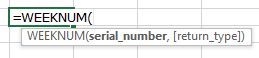For Example: - If we have 1st January, 2015 then week number will be 1.

Let’s take an example and understand:-

We have a date range in Excel worksheet, and we want to return week number for all dates.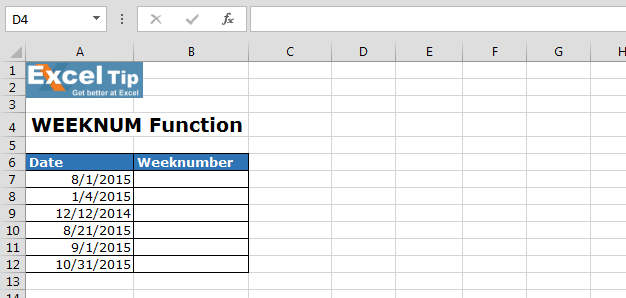To return the week number, follow below given steps:-

• Enter the formula in cell B7
• =WEEKNUM(select date cell then , select return type in numbers eg:- 1,2,3..)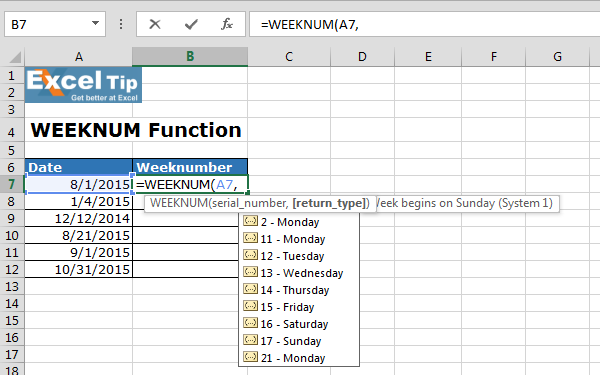Note: - Return type is optional, it is a number which indicates the day the week begins.

• =WEEKNUM(A7,1)
• Press Enter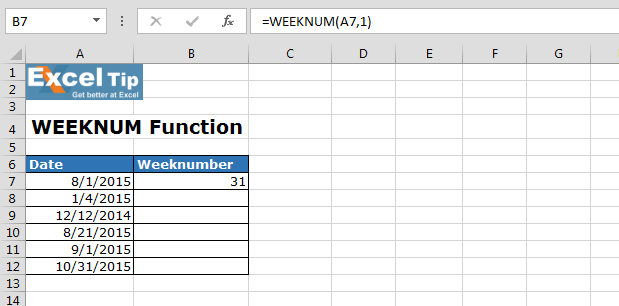• Copying down the formula from cell B7 to range B8:B12will get the desired result.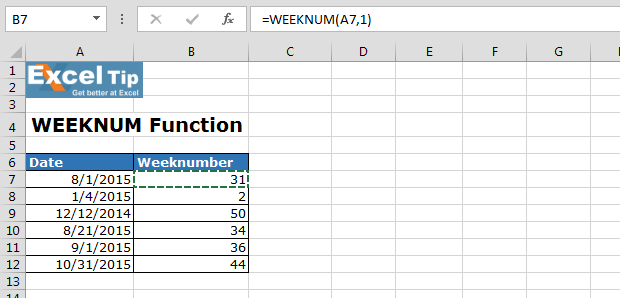Note: - In this example, week is starting from the 1st day and Sunday.

In this way, we can easily return the week number to a date in Microsoft Excel.